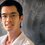# Solve using basic Geometry!!(Challenge!)

In triangle $ABC,AM$ is the median such that $\angle MAB = 105^{\circ}$ and $\angle B = \angle MAC$,find $\angle B$? The problem looks simple but my challenge is to solve it using basic geometry(congruency,similarity etc) and not trigonometry.Note by Kishan K
6 years, 10 months ago

This discussion board is a place to discuss our Daily Challenges and the math and science related to those challenges. Explanations are more than just a solution — they should explain the steps and thinking strategies that you used to obtain the solution. Comments should further the discussion of math and science.

When posting on Brilliant:

• Use the emojis to react to an explanation, whether you're congratulating a job well done , or just really confused .
• Ask specific questions about the challenge or the steps in somebody's explanation. Well-posed questions can add a lot to the discussion, but posting "I don't understand!" doesn't help anyone.
• Try to contribute something new to the discussion, whether it is an extension, generalization or other idea related to the challenge.

MarkdownAppears as
*italics* or _italics_ italics
**bold** or __bold__ bold
- bulleted- list
• bulleted
• list
1. numbered2. list
1. numbered
2. list
Note: you must add a full line of space before and after lists for them to show up correctly
paragraph 1paragraph 2

paragraph 1

paragraph 2

[example link](https://brilliant.org)example link
> This is a quote
This is a quote
    # I indented these lines
# 4 spaces, and now they show
# up as a code block.

print "hello world"
# I indented these lines
# 4 spaces, and now they show
# up as a code block.

print "hello world"
MathAppears as
Remember to wrap math in $$ ... $$ or $ ... $ to ensure proper formatting.
2 \times 3 $2 \times 3$
2^{34} $2^{34}$
a_{i-1} $a_{i-1}$
\frac{2}{3} $\frac{2}{3}$
\sqrt{2} $\sqrt{2}$
\sum_{i=1}^3 $\sum_{i=1}^3$
\sin \theta $\sin \theta$
\boxed{123} $\boxed{123}$

Sort by:

Great problem..man I haven't done a geometric proofs in such a long time, reminds me of the AoPS days:)

Here's a solution:

First of all since the triangles $CAM,CBA$ share the same angle $\angle C$ and $\angle B=\angle MAC$, they are similar! Therefore their corresponding sides are proportional which gives: $\frac {CM}{AC}=\frac {AC}{CB} \Rightarrow AC^2=CM*AB=2CM^2 \Rightarrow \frac {AB}{AM}=\frac {AC}{CM}=\sqrt {2}$.

So now the problem has been simplified to a more straight forward question: Given a triangle $BAM$ with $\angle BAM=105$ and $\frac {AB}{AM}=\sqrt {2}$, find $\angle B$ (Note that this triangle is "similarly" unique).

To proceed, let's get a bit "creative", what does the ratio of two sides equal to root 2 remind us of? A $45-45-90$ triangle? Let's try to construct one in this diagram! Construct a point $N$ on the same side as $M$ wrt $AB$ such that $\angle ANB=90, \angle BAN=45$. This means that $\angle NAM=105-45=60$. Since $AN=\frac {AB}{\sqrt {2}}=AM$, we obtain that $AMN$ is an equilateral triangle. Furthermore, we have $BN=AN=AM=NM$. Since $\angle BNM=90+60=150$, therefore $\angle MBN=\frac {180-150}{2}=15$ which means that $\angle B=45-15=30$! and that's our answer.

- 6 years, 10 months ago

Being too much exited, you made the answer factorial 30=$30!$. Ha ha ha. Good job.

- 6 years, 10 months ago

Nice solution! I also found that $\frac{AB}{AM}=\sqrt{2}$ using the same similarity, but finished with a "trick" solution.

Motivation: Note that $\frac{\sin \angle AMB}{\sin \angle ABM }=\sqrt{2}$, thus you need two angles whose sum is $75^\circ$ and whose ratio of sines is $\sqrt{2}$ ...yes! 45° and 30°.

Proof. Consider a triangle $B'A'M'$ such that $\angle A'B'M'=30^\circ$ and $\angle B'M'A'=45^\circ$, note that $\frac{A'B'}{A'M'}=\frac{\sin 45^\circ}{\sin 30^\circ}$, then $\frac{A'B'}{A'M'}=\frac{AB}{AM}$ and $\angle B'A'M'=\angle BAM=105^\circ$, i.e. triangles $BAM$ and $B'A'M'$ are similar and we are done.

- 6 years, 10 months ago

For another good geometry problem go to https://brilliant.org/discussions/thread/geometry-problemagain-a-challenge/

- 6 years, 10 months ago

Hmm I got that no triangle exists... (by angle chasing and similar triangles)

EDIT: Oops I misread my paper, I'll post a solution soon.

- 6 years, 10 months ago

I too got such a solution, and whats more, i got that the sum of angles of triangle ABC is greater than 210 degrees.all i did then was abandon this approach and look for another set of triangles where basic geo could be used!!and Lo it was there!!

- 6 years, 10 months ago

Great post dude. Need more of this kind to refresh minds

- 6 years, 10 months ago